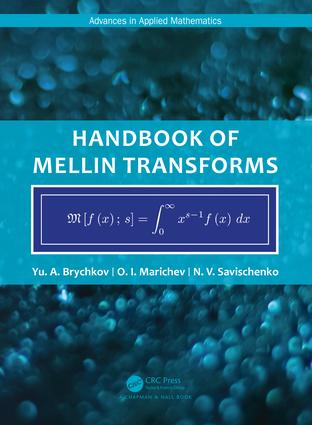Handbook of Mellin Transforms

1st Edition

Chapman and Hall/CRC

587 pages

Hardback: 9781138353350
pub: 2018-10-02
\$299.00
x
eBook (VitalSource) : 9780429434259
pub: 2018-10-10
from \$149.50

FREE Standard Shipping!

Description

The Mellin transformation is widely used in various problems of pure and applied mathematics, in particular, in the theory of differential and integral equations and the theory of Dirichlet series. It is found in extensive applications in mathematical physics, number theory, mathematical statistics, theory of asymptotic expansions, and especially, in the theory of special functions and integral transformations. It is essentially used in algorithms of integration in computer algebra systems.

Since the majority of integrals encountered in applications can be reduced to the form of the corresponding Mellin transforms with specific parameters, this handbook can also be used for definite and indefinite integrals. By changes in variables, the Mellin transform can be turned into the Fourier and Laplace transforms.

The appendices contain formulas of connection with other integral transformations, and an algorithm for determining regions of convergence of integrals.

The Handbook of Mellin Transforms will be of interest and useful to all researchers and engineers who use mathematical methods. It will become the main source of formulas of Mellin transforms, as well as indefinite and definite integrals.

General Formulas

Elementary Functions

Special Functions

Appendix I: Some properties of the Mellin transforms

Appnedix II: Condtions of convergence

Yu.A. Brychkov, https://en.wikipedia.org/wiki/Yuri_Aleksandrovich_Brychkov, graduated from the Lomonosov Moscow State University. He was a post-graduate student in the Mathematical Institute of the Russian Academy of Sciences and has been at the Dorodnitsyn Computing Center of the Russian Academy of Sciences since 1969. He has published about 100 publications including 2 books and 7 handbooks in CRC (Gordon and Breach) including 5 volumes of "Integrals and Series" (together with A.P.Prudnikov and O.I.Marichev).

O.I. Marichev, https://en.wikipedia.org/wiki/Oleg_Marichev, Graduated from the Belorussian State University, he received the D.Sc. degree (Habilitation) in mathematics from the University of Jena, Germany. In 1991, he started working with Stephen Wolfram on Mathematica, developing integration and mathematical functions. He has authored about 70 publications, is an author and a co-author of 10 books and the well-known Wolfram Functions site http://- functions.wolfram.com/ with over 307,000 formulas (http://functions.wolfram.com/About/developers.html). His books include "Fractional Integrals and Derivatives. Theory and Applications." (Samko S.G., Kilbas A.A., Marichev O.I., 1987, 1993).

N.V.Savischenko, graduated from the Novosibirsk State University, and has been at the Military Telecommunications Academy since 1987, publishing nearly 100 articles and a book.